true# Krishna Agarwal

## Msc physics student want to learn and Teach physic...

JP Nagar 1st Phase, Bangalore, India - 560078.Verified13 Students

Referral Discount: Get ₹ 500 off when you make a payment to start classes. Get started by Booking a Demo.

Details verified of Krishna Agarwal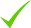IdentityEducation

Know how UrbanPro verifies Tutor details

Identity is verified based on matching the details uploaded by the Tutor with government databases.

## Overview

I am an experienced, private tutor for 1 year for mathematics and physics for C.B.S.E and I.C.S.E. Love to teach and explain my students in a easy way. Currently i'm following my passion of studying high level physics,because this subject always give you new things to think about and i would like to give my students same feeling about this subject. I did my B.Sc from Utkal university Bhubneshwar,Odisha as Physics(hons.) with 85% in physics and 70.33% as aggregate.

## Languages Spoken

Hindi Mother Tongue (Native)

English Proficient

## Education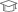utkal university 2018

Bachelor of Science (B.Sc.)

JP Nagar 1st Phase, Bangalore, India - 560078

## Verified Info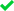ID VerifiedEducation VerifiedPhone VerifiedEmail Verified

Report this Profile

Is this listing inaccurate or duplicate? Any other problem?

Type the letters as shown below *

Please enter the letters as show below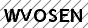## Teaches

Class 9 Tuition

Class LocationOnline Classes (Video Call via UrbanPro LIVE)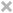Student's HomeTutor's Home

Board

CBSE, ICSE

CBSE Subjects taught

Mathematics, Science

ICSE Subjects taught

Chemistry, Physics, Mathematics

Taught in School or College

No

Class 10 Tuition

Class LocationOnline Classes (Video Call via UrbanPro LIVE)Student's HomeTutor's Home

Board

CBSE, ICSE

CBSE Subjects taught

Mathematics, Science

ICSE Subjects taught

Chemistry, Physics, Mathematics

Taught in School or College

No

Class 11 Tuition

Class LocationOnline Classes (Video Call via UrbanPro LIVE)Student's HomeTutor's Home

Board

CBSE

CBSE Subjects taught

Physics, Mathematics

Taught in School or College

No

Class 12 Tuition

Class LocationOnline Classes (Video Call via UrbanPro LIVE)Student's HomeTutor's Home

Board

CBSE

CBSE Subjects taught

Physics

Taught in School or College

No

Gauss Law

## Reviews (8)

5 out of 5 8 reviews

Krishna Agarwal https://s3-ap-southeast-1.amazonaws.com/tv-prod/member/photo/6814850-small.jpg JP Nagar 1st Phase
5.0058
Krishna Agarwal
G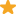"His teaching style is very good. He has good knowledge about subject. He is very kind with student. I scored good marks in every exam. "

Krishna Agarwal
J"Explains problems in simplest manners, Very punctual with timings. Knowledgeable and energetic teacher. "

Krishna Agarwal
H"Knowledgeable person and teaching style are very good. I learnt a lot from him. He has a number of ways to make things understandable. I think which is the most important thing. Because every student has a different mindset and capabilities. "

Krishna Agarwal
A"Deep understanding of the subjects along with an easy and simple way of teaching. Definitely one of the best teacher I have ever got the opportunity to learn from. "

Have you attended any class with Krishna? Write a Review

## FAQs

1. Which school boards of Class 10 do you teach for?

CBSE and ICSE

2. Do you have any prior teaching experience?

No

3. Which classes do you teach?

I teach Class 10 Tuition, Class 11 Tuition, Class 12 Tuition and Class 9 Tuition Classes.

4. Do you provide a demo class?

Yes, I provide a free demo class.

5. How many years of experience do you have?

I have been teaching for less than a year.

Consider X:Y:Z=ai.e X/Y=aY/Z=aand Z/X=anow lets back to our question 5(X^2+Y^2+Z^2)=4(XY+YZ+ZX)divide both side by XYZ5{(X/YZ)+(Y/XZ)+(Z/XY)}=4(1/X+1/Y+1/Z)from our previous assumption5(a/Z+a/X+a/Y)=4(1/X+1/Y+1/Z)5a(1/X+1/Y+1/Z)=4(1/X+1/Y+1/Z)5a=4a=4/5so X:Y:X=4/5 ...more

Consider X:Y:Z=a
i.e X/Y=a
Y/Z=a
and Z/X=a
now lets back to our question
5(X^2+Y^2+Z^2)=4(XY+YZ+ZX)
divide both side by XYZ
5{(X/YZ)+(Y/XZ)+(Z/XY)}=4(1/X+1/Y+1/Z)
from our previous assumption
5(a/Z+a/X+a/Y)=4(1/X+1/Y+1/Z)
5a(1/X+1/Y+1/Z)=4(1/X+1/Y+1/Z)
5a=4
a=4/5
so X:Y:X=4/5

Dislike Bookmark

Consider X:Y:Z=ai.e X/Y=aY/Z=aand Z/X=anow lets back to our question 5(X^2+Y^2+Z^2)=4(XY+YZ+ZX)divide both side by XYZ5{(X/YZ)+(Y/XZ)+(Z/XY)}=4(1/X+1/Y+1/Z)from our previous assumption5(a/Z+a/X+a/Y)=4(1/X+1/Y+1/Z)5a(1/X+1/Y+1/Z)=4(1/X+1/Y+1/Z)5a=4a=4/5so X:Y:X=4/5 ...more

Consider X:Y:Z=a
i.e X/Y=a
Y/Z=a
and Z/X=a
now lets back to our question
5(X^2+Y^2+Z^2)=4(XY+YZ+ZX)
divide both side by XYZ
5{(X/YZ)+(Y/XZ)+(Z/XY)}=4(1/X+1/Y+1/Z)
from our previous assumption
5(a/Z+a/X+a/Y)=4(1/X+1/Y+1/Z)
5a(1/X+1/Y+1/Z)=4(1/X+1/Y+1/Z)
5a=4
a=4/5
so X:Y:X=4/5

Dislike Bookmark

Yes, it has very small weight.
Dislike Bookmark

Answered on 14/05/2020 Learn Unit III: Calculus/Applications of Derivatives +1

You can use Zoom.
Dislike Bookmark

## Teaches

Class 9 Tuition

Class LocationOnline Classes (Video Call via UrbanPro LIVE)Student's HomeTutor's Home

Board

CBSE, ICSE

CBSE Subjects taught

Mathematics, Science

ICSE Subjects taught

Chemistry, Physics, Mathematics

Taught in School or College

No

Class 10 Tuition

Class LocationOnline Classes (Video Call via UrbanPro LIVE)Student's HomeTutor's Home

Board

CBSE, ICSE

CBSE Subjects taught

Mathematics, Science

ICSE Subjects taught

Chemistry, Physics, Mathematics

Taught in School or College

No

Class 11 Tuition

Class LocationOnline Classes (Video Call via UrbanPro LIVE)Student's HomeTutor's Home

Board

CBSE

CBSE Subjects taught

Physics, Mathematics

Taught in School or College

No

Class 12 Tuition

Class LocationOnline Classes (Video Call via UrbanPro LIVE)Student's HomeTutor's Home

Board

CBSE

CBSE Subjects taught

Physics

Taught in School or College

No

## Answers by Krishna Agarwal (4)

Consider X:Y:Z=ai.e X/Y=aY/Z=aand Z/X=anow lets back to our question 5(X^2+Y^2+Z^2)=4(XY+YZ+ZX)divide both side by XYZ5{(X/YZ)+(Y/XZ)+(Z/XY)}=4(1/X+1/Y+1/Z)from our previous assumption5(a/Z+a/X+a/Y)=4(1/X+1/Y+1/Z)5a(1/X+1/Y+1/Z)=4(1/X+1/Y+1/Z)5a=4a=4/5so X:Y:X=4/5 ...more

Consider X:Y:Z=a
i.e X/Y=a
Y/Z=a
and Z/X=a
now lets back to our question
5(X^2+Y^2+Z^2)=4(XY+YZ+ZX)
divide both side by XYZ
5{(X/YZ)+(Y/XZ)+(Z/XY)}=4(1/X+1/Y+1/Z)
from our previous assumption
5(a/Z+a/X+a/Y)=4(1/X+1/Y+1/Z)
5a(1/X+1/Y+1/Z)=4(1/X+1/Y+1/Z)
5a=4
a=4/5
so X:Y:X=4/5

Dislike Bookmark

Consider X:Y:Z=ai.e X/Y=aY/Z=aand Z/X=anow lets back to our question 5(X^2+Y^2+Z^2)=4(XY+YZ+ZX)divide both side by XYZ5{(X/YZ)+(Y/XZ)+(Z/XY)}=4(1/X+1/Y+1/Z)from our previous assumption5(a/Z+a/X+a/Y)=4(1/X+1/Y+1/Z)5a(1/X+1/Y+1/Z)=4(1/X+1/Y+1/Z)5a=4a=4/5so X:Y:X=4/5 ...more

Consider X:Y:Z=a
i.e X/Y=a
Y/Z=a
and Z/X=a
now lets back to our question
5(X^2+Y^2+Z^2)=4(XY+YZ+ZX)
divide both side by XYZ
5{(X/YZ)+(Y/XZ)+(Z/XY)}=4(1/X+1/Y+1/Z)
from our previous assumption
5(a/Z+a/X+a/Y)=4(1/X+1/Y+1/Z)
5a(1/X+1/Y+1/Z)=4(1/X+1/Y+1/Z)
5a=4
a=4/5
so X:Y:X=4/5

Dislike Bookmark

Yes, it has very small weight.
Dislike Bookmark

Answered on 14/05/2020 Learn Unit III: Calculus/Applications of Derivatives +1

You can use Zoom.
Dislike Bookmark

Krishna Agarwal describes himself as Msc physics student want to learn and Teach physics. He conducts classes in Class 10 Tuition, Class 11 Tuition and Class 12 Tuition. Krishna is located in JP Nagar 1st Phase, Bangalore. Krishna takes Regular Classes- at his Home and Online Classes- via online medium. Krishna has completed Bachelor of Science (B.Sc.) from utkal university in 2018. He is well versed in English and Hindi. Krishna has got 8 reviews till now with 100% positive feedback.

•• Want to learn from Krishna?

• Book a Demo
X

Recommended Profiles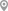JP Nagar 1st Phase, BangaloreJP Nagar 1st Phase, BangaloreJP Nagar 1st Phase, BangaloreJP Nagar 1st Phase, BangaloreJP Nagar 1st Phase, BangaloreJP Nagar 1st Phase, Bangalore

1500/1500Certified